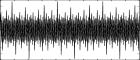## GEN34

GEN34 — Generate composite waveforms by mixing simple sinusoids.

## Description

These routines generate composite waveforms by mixing simple sinusoids, similarly to GEN09, but the parameters of the partials are specified in an already existing table, which makes it possible to calculate any number of partials in the orchestra.

The difference between GEN33 and GEN34 is that GEN33 uses inverse FFT to generate output, while GEN34 is based on the algorithm used in oscils opcode. GEN33 allows integer partials only, and does not support power of two plus 1 table size, but may be significantly faster with a large number of partials. On the other hand, with GEN34, it is possible to use non-integer partial numbers and extended guard point, and this routine may be faster if there is only a small number of partials (note that GEN34 is also several times faster than GEN09, although the latter may be more accurate).

## Syntax

`f # time size  34  src nh scl [fmode]`

## Initialization

size -- number of points in the table. Must be power of two or a power of two plus 1.

src -- source table number. This table contains the parameters of each partial in the following format:

stra, pna, phsa, strb, pnb, phsb, ...

the parameters are:

• stra, strb, etc.: relative strength of partials. The actual amplitude depends on the value of scl, or normalization (if enabled).

• pna, pnb, etc.: partial number, or frequency, depending on fmode (see below); zero and negative values are allowed, however, if the absolute value of the partial number exceeds (size / 2), the partial will not be rendered.

• phsa, phsb, etc.: initial phase, in the range 0 to 1.

Table length (not including the guard point) should be at least 3 * nh. If the table is too short, the number of partials (nh) is reduced to (table length) / 3, rounded towards zero.

nh -- number of partials. Zero or negative values are allowed, and result in an empty table (silence). The actual number may be reduced if the source table (src) is too short, or some partials have too high frequency.

scl -- amplitude scale.

fmode (optional, default = 0) -- a non-zero value can be used to set frequency in Hz instead of partial numbers in the source table. The sample rate is assumed to be fmode if it is positive, or -(sr * fmode) if any negative value is specified.

## Examples

Here is an example of the GEN34 routine. It uses the file gen34.csd.

Example 1297. Example of the gen34 generator.

See the sections Real-time Audio and Command Line Flags for more information on using command line flags.

```<CsoundSynthesizer>
<CsOptions>
; Select audio/midi flags here according to platform
-odac      ;;;realtime audio out
; For Non-realtime ouput leave only the line below:
; -o gen34.wav -W ;;; for file output any platform
</CsOptions>
<CsInstruments>

sr = 44100
ksmps = 32
nchnls = 2
0dbfs  = 1

instr 1	; partials 1, 4, 7, 10, 13, 16, etc. with base frequency of 400 Hz

ibsfrq  =  400
inumh   =  int(1.5 + sr * 0.5 / (3 * ibsfrq))					; estimate number of partials
isrcln  =  int(0.5 + exp(log(2) * int(1.01 + log(inumh * 3) / log(2))))		; source table length
itmp    ftgen 1, 0, isrcln, -2, 0						; create empty source table
ifpos   =  0
ifrq    =  ibsfrq
inumh   =  0
l1:
tableiw ibsfrq / ifrq, ifpos, 1						; amplitude
tableiw ifrq, ifpos + 1, 1						; frequency
tableiw 0, ifpos + 2, 1							; phase
ifpos   =  ifpos + 3
ifrq    =  ifrq + ibsfrq * 3
inumh   =  inumh + 1
if (ifrq < (sr * 0.5)) igoto l1

itmp    ftgen 2, 0, 262144, -34, 1, inumh, 1, -1				; store output in ftable 2 (size = 262144)
asig    poscil .5, ibsfrq, itmp
outs asig, asig

endin
</CsInstruments>
<CsScore>

i 1 0 2

e
</CsScore>
</CsoundSynthesizer>
```

This is the diagram of the waveform generated by GEN34, as used in the example:f 2 0 262144 -34 1 inumh 1 -1